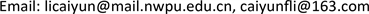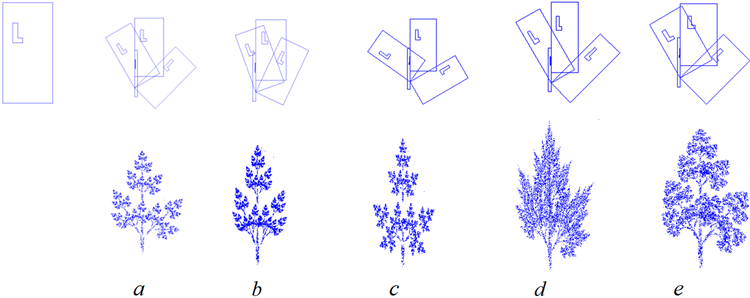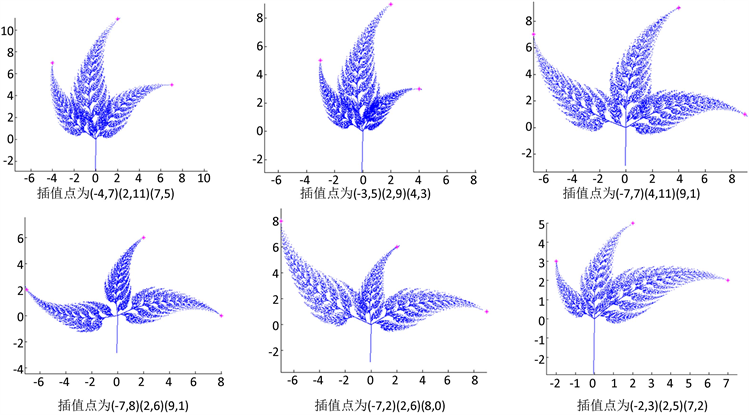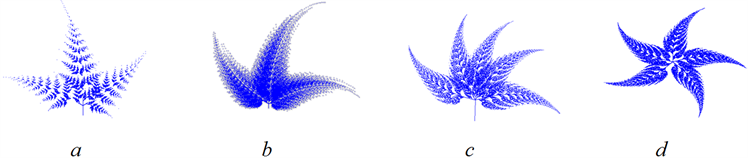﻿ 基于迭代函数系统的分形植物模拟 Simulation of Fractal Plant Based on Iteration Function System

Vol.07 No.01(2018), Article ID:23616,11 pages
10.12677/AAM.2018.71016

Simulation of Fractal Plant Based on Iteration Function System

Caiyun Li, Hongchan Zheng, Zengyao Lin

Department of Applied Mathematics, Northwestern Polytechnical University, Xi’an ShanxiReceived: Dec. 23rd, 2017; accepted: Jan. 23rd, 2018; published: Jan. 30th, 2018ABSTRACT

In this paper, based on the introduction of the basic theory of Iterative Function System(IFS), the first application of IFS code to a given image can reveal the shrinkage characteristics of internal affine transformation. The influence of the numbers and parameters of affine transformations on the generation of fractal tree is analyzed. Thus it can generate fractal trees with different morphologies by modifying the IFS code. Using pseudo-affine transformation to achieve the interpolation of the attractor image, the triangular maple leaves and the pentagon maple leaves are generated. Meanwhile, controllable fractal leaves can be obtained through adjusting interpolation points. Finally, some examples of fractal images are given with Matlab.

Keywords:Fractal, Plant Simulation, Pseudo-Affine Transformation, Maple Leaves1. 引言

2. 预备知识

$W\left[\begin{array}{c}x\\ y\end{array}\right]=\left[\begin{array}{cc}a& b\\ c& d\end{array}\right]\left[\begin{array}{c}x\\ y\end{array}\right]+\left[\begin{array}{c}e\\ f\end{array}\right],$

$x\in {R}^{2}$ 时，上式常改写为

$W\left(x\right)=Ax+t,$

$\rho \left(f\left(x\right),f\left(y\right)\right)\le s\rho \left(x,y\right),\text{\hspace{0.17em}}\forall x,y\in X,$

$s$ 称为 $f$ 的压缩因子。

$W\left(B\right)=\underset{n=1}{\overset{N}{\cup }}{W}_{n}\left(B\right),\text{\hspace{0.17em}}\forall B\in H\left(X\right),$

$W$ 是完备空间 $\left(H\left(X\right),h\left(\rho \right)\right)$ 上具有压缩因子 $s$ 的压缩映射，即

$h\left(W\left(A\right),W\left(B\right)\right)\le sh\left(A,B\right),\text{\hspace{0.17em}}\forall A,B\in H\left(X\right),$

$P=W\left(P\right)=\underset{n=1}{\overset{N}{\cup }}{W}_{n}\left(P\right),$

$P=\underset{n\to \infty }{\mathrm{lim}}{W}^{n}\left(B\right),\forall B\in H\left(X\right)$ 。不动点集 $P\in H\left(X\right)$ 被称为IFS的吸引子。

3. 不同形态分形树的生成

3.1. 简单的变换

$\left[\begin{array}{cc}a& b\\ c& d\end{array}\right]=\left[\begin{array}{cc}r\mathrm{cos}\theta & -s\mathrm{sin}\phi \\ r\mathrm{sin}\theta & s\mathrm{cos}\phi \end{array}\right]$

$\begin{array}{l}r=\sqrt{{a}^{2}+{c}^{2}}\\ \theta =\mathrm{arccos}\left(\frac{a}{\sqrt{{a}^{2}+{c}^{2}}}\right)\end{array}$初始图像 缩放 错切 旋转 反射

Figure 1. Basic transformation of graphics

3.2. 分形树的算法实现

$\sum {p}_{n}=1,\text{\hspace{0.17em}}0<{p}_{n}<1,\text{\hspace{0.17em}}\left(n=1,2,\cdots ,N\right),$

${p}_{n}=\frac{|{A}_{n}|}{\sum _{n=1}^{N}|{A}_{n}|}=\frac{|{a}_{n}{d}_{n}-{b}_{n}{c}_{n}|}{\sum _{n=1}^{N}|{a}_{n}{d}_{n}-{b}_{n}{c}_{n}|},$

(1) 定义IFSP为 $\left\{X;{W}_{n},{p}_{n},n=1,2,\cdots ,N\right\}$ ，设定初始点 $\left({x}_{0},{y}_{0}\right)$ 和迭代次数 $level$

(2) 利用rand()函数生成在区间 $\left[0,1\right]$ 的随机数 $R$ ，以概率 ${p}_{n}$ 选取仿射变换 ${W}_{n}$

(3) 以 ${W}_{n}$ 作用点 $\left({x}_{0},{y}_{0}\right)$ ，得到新坐标 $\left({x}_{1},{y}_{1}\right)$

(4) 令 ${x}_{0}={x}_{1},{y}_{0}={y}_{1}$ 并在屏幕上打出 $\left({x}_{0},{y}_{0}\right)$

(5) 重返第(2)步，进行下一次的迭代，直到迭代次数大于 $level$ 为止。Figure 2. Fractal trees of affine transform with different parameters. The first figure of the first line is the given original image, the last five figures are the images after the first affine transformation of the initial image, and the second line corresponds to the attractor of the IFS codeFigure 3. Fractal trees of affine transform with different numbers. The first figure of the first line is the given original image, the last four figures are the images after the first affine transformation of the initial image, and the second line corresponds to the attractor of the IFS code

4. 采用拟仿射变换生成三角枫叶、五角枫叶

4.1. 拟仿射变换

IFS是以仿射变换为框架，经过迭代生成的，但迭代生成的整体分形图却不能简单地使用几何学上的仿射变换的方法实现。我们将分形图整体平移、缩放、旋转、错切等几何变换称为拟仿射变换。其原因在于分形图是一组仿射变换所确定的不动点集，对这些不动点集的拟仿射变换过程就是使这些不动点集从一个状态换到另一个状态的过程，变换后分形图的结构、状态和点的分布密度仍然受IFS码的控制，即在具体算法中，对迭代生成的点进行平移、旋转、缩放、错切等变换操作之后，必须将变换之前的点作为下一次迭代的初值，从而就可以实现分形图整体的仿射变换。在下节中介绍从分形图的拟仿射变换迭代产生的拟仿射变换模型，进而实现分形图的拟仿射变换。

4.2. 分形图的拟仿射变换

${X}_{k}={A}_{i}×{X}_{k-1}+{E}_{i},$

$\left[\begin{array}{c}{x}_{k}\\ {y}_{k}\end{array}\right]=\left[\begin{array}{cc}{a}_{i}& {b}_{i}\\ {c}_{i}& {d}_{i}\end{array}\right]×\left[\begin{array}{c}{x}_{k-1}\\ {y}_{k-1}\end{array}\right]+\left[\begin{array}{c}{e}_{i}\\ {f}_{i}\end{array}\right].$

(1) 分形图的平移变换

$\left[\begin{array}{c}{x}_{k}\\ {y}_{k}\end{array}\right]=\left[\begin{array}{cc}{a}_{i}& {b}_{i}\\ {c}_{i}& {d}_{i}\end{array}\right]\left[\begin{array}{c}{x}_{k-1}\\ {y}_{k-1}\end{array}\right]-\left[\begin{array}{cc}{a}_{i}& {b}_{i}\\ {c}_{i}& {d}_{i}\end{array}\right]\left[\begin{array}{c}{d}_{x}\\ {d}_{y}\end{array}\right]+\left[\begin{array}{c}{e}_{i}\\ {f}_{i}\end{array}\right]+\left[\begin{array}{c}{d}_{x}\\ {d}_{y}\end{array}\right].$

(2) 分形图的旋转变换

$\left[\begin{array}{c}{x}_{k}\\ {y}_{k}\end{array}\right]=\left[\begin{array}{cc}\mathrm{cos}\theta & -\mathrm{sin}\theta \\ \mathrm{sin}\theta & \mathrm{cos}\theta \end{array}\right]\left[\begin{array}{cc}{a}_{i}& {b}_{i}\\ {c}_{i}& {d}_{i}\end{array}\right]\left[\begin{array}{cc}\mathrm{cos}\theta & \mathrm{sin}\theta \\ -\mathrm{sin}\theta & \mathrm{cos}\theta \end{array}\right]\left[\begin{array}{c}{x}_{k-1}\\ {y}_{k-1}\end{array}\right]+\left[\begin{array}{cc}\mathrm{cos}\theta & -\mathrm{sin}\theta \\ \mathrm{sin}\theta & \mathrm{cos}\theta \end{array}\right]\left[\begin{array}{c}{e}_{i}\\ {f}_{i}\end{array}\right].$

(3) 分形图的缩放变换

$\left[\begin{array}{c}{x}_{k}\\ {y}_{k}\end{array}\right]=\left[\begin{array}{cc}{K}_{x}& 0\\ 0& {K}_{y}\end{array}\right]\left[\begin{array}{cc}{a}_{i}& {b}_{i}\\ {c}_{i}& {d}_{i}\end{array}\right]\left[\begin{array}{cc}1/{K}_{x}& 0\\ 0& 1/{K}_{y}\end{array}\right]\left[\begin{array}{c}{x}_{k-1}\\ {y}_{k-1}\end{array}\right]+\left[\begin{array}{cc}{K}_{x}& 0\\ 0& {K}_{y}\end{array}\right]\left[\begin{array}{c}{e}_{i}\\ {f}_{i}\end{array}\right].$

(4) 分形图的错切变换

$\left[\begin{array}{c}{x}_{k}\\ {y}_{k}\end{array}\right]=\left[\begin{array}{cc}1& {S}_{x}\\ 0& 1\end{array}\right]\left[\begin{array}{cc}{a}_{i}& {b}_{i}\\ {c}_{i}& {d}_{i}\end{array}\right]\left[\begin{array}{cc}1& -{S}_{x}\\ 0& 1\end{array}\right]\left[\begin{array}{c}{x}_{k-1}\\ {y}_{k-1}\end{array}\right]+\left[\begin{array}{cc}1& {S}_{x}\\ 0& 1\end{array}\right]\left[\begin{array}{c}{e}_{i}\\ {f}_{i}\end{array}\right].$

$\left[\begin{array}{c}{x}_{k}\\ {y}_{k}\end{array}\right]=\left[\begin{array}{cc}1& 0\\ {S}_{y}& 1\end{array}\right]\left[\begin{array}{cc}{a}_{i}& {b}_{i}\\ {c}_{i}& {d}_{i}\end{array}\right]\left[\begin{array}{cc}1& 0\\ -{S}_{y}& 1\end{array}\right]\left[\begin{array}{c}{x}_{k-1}\\ {y}_{k-1}\end{array}\right]+\left[\begin{array}{cc}1& 0\\ {S}_{y}& 1\end{array}\right]\left[\begin{array}{c}{e}_{i}\\ {f}_{i}\end{array}\right].$

$\alpha =\mathrm{arccos}\left(\frac{\left({a}_{1}^{2}+{b}_{1}^{2}\right)+\left({a}_{2}^{2}+{b}_{2}^{2}\right)-\left({\left({a}_{1}-{a}_{2}\right)}^{2}+{\left({b}_{1}-{b}_{2}\right)}^{2}\right)}{2×\sqrt{{a}_{1}^{2}+{b}_{1}^{2}}×\sqrt{{a}_{2}^{2}+{b}_{2}^{2}}}\right),$

$L=\frac{\sqrt{{a}_{2}^{2}+{b}_{2}^{2}}}{\sqrt{{a}_{1}^{2}+{b}_{1}^{2}}},$

4.3. 可控枫叶的算法实现

(1) 给定初始插值点 $\left({a}_{2},{b}_{2}\right),\left({a}_{3},{b}_{3}\right),\cdots ,\left({a}_{m},{b}_{m}\right)$

(2) 用上节给出的随机迭代算法生成目标树叶；

(3) 对目标树叶运用拟仿射变换模型，调用m次旋转变换以及缩放变换。Figure 4. The triangular maple leaves generated by the given three interpolation pointsFigure 5. Fractal leaves. The first two figures are triangular leaves by changing the IFS code, and the last two are pentagonal leaves by changing the number of leaves

5. 结论

Simulation of Fractal Plant Based on Iteration Function System[J]. 应用数学进展, 2018, 07(01): 128-138. http://dx.doi.org/10.12677/AAM.2018.71016

1. 1. Prusinkiewicz, P., Hammel, M. and Mjolsness, E. (1993) Animation of Plant Development. Computer Graphics, 27, 351-360.
https://doi.org/10.1145/166117.166161

2. 2. Prusinkiewicz, P. and Lindenmayer, A. (1990) The Algo-rithmic Beauty of Plants. Springer-Verlag, New York.
https://doi.org/10.1007/978-1-4613-8476-2

3. 3. 李庆忠, 韩金殊. 基于IFS的树木形态模拟方法[J]. 计算机辅助工程, 2004, 13(4): 20-24.

4. 4. 肖海蓉. 基于IFS分形算法的树木形态分析和实现[J]. 计算机仿真, 2011, 28(6): 274-279.

5. 5. Zhang, H.Q. and Liu, M. (2009) Tree Growth Simulation Method Based on Improved IFS Algo-rithm. International Conference on Computer Intelligence and Software Engineering, 1-5.
https://doi.org/10.1109/CISE.2009.5365658

6. 6. 袁琪, 周淑秋, 郭新宇. 粒子系统在虚拟作物生长中的应用研究[J]. 微计算机信息, 2007, 23(21): 262-264.

7. 7. 宋万寿, 赖建伟. 基于粒子系统方法的焰火及树木模拟[J]. 计算机辅助设计与图形学学报, 1996, 8(4): 254-258.

8. 8. 朱华, 姬翠翠. 分形理论及其应用[M]. 北京: 科学出版社, 2011.

9. 9. 郝小琴. 森林景物的三维迭代函数系统建模技术的研究[J]. 计算机学报, 1999, 22(7): 768-773.

10. 10. 仲兰芬, 王琰, 程磊. 三维分形树木IFS生成算法[J]. 沈阳理工大学学报, 2005, 24(1): 28-31.

11. 11. 潘陆益. IFS分形图拟仿射变换模型及其实现[J]. 计算机系统应用, 2008, 17(1): 83-84.

12. 12. 韩江萍, 周敏, 郑红婵, 等. 采用拟仿射变换进行分形树模拟[J]. 计算机工程和设计, 2012, 33(2): 700-704.

13. 13. 仲兰芬, 王文忠. 由叶轮廓生成叶脉的树叶建模与绘制[J]. 小型微型计算机系统, 2016, 37(5): 1017-1021.

14. 14. 赵健, 雷蕾, 蒲小勤. 分形理论及其在信号处理中的应用[M]. 北京: 清华大学出版社, 2008.

15. 15. 海因茨•奥托•佩特根, 哈特穆特•于尔根斯, 迪特马尔•绍柏. 混沌与分形[M]. 北京: 国防工业出版社, 2008.

16. 16. 楼顺天, 胡昌华, 张伟. 基于MATLAB的系统分析与设计—模糊系统[M]. 西安: 西安电子科技大学出版社, 2001.Table 1. Affine transformation parameters of Figure 2(a)Table 2. Affine transformation parameters of Figure 3(a)Table 3. Affine transformation parameters of Figure 3(b)Table 4. Affine transformation parameters of Figure 3(c)Table 5. Affine transformation parameters of Figure 3(d)Table 6. Affine transformation parameters of Figure 4

1. 打开知网页面http://kns.cnki.net/kns/brief/result.aspx?dbPrefix=WWJD

2. 打开知网首页http://cnki.net/